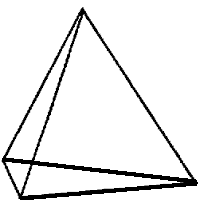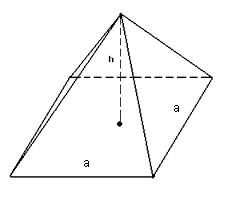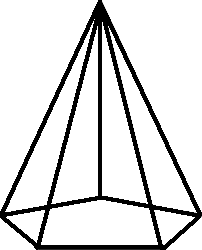Pyramid Calculator

Calculate the various properties of various Pyramids for given values.
Regular Triangular Pyramid
 Enter the Apothem Lengt : Enter the Side Length: Enter the Slant height: Enter the Height:

 Area of Base = Surface Area of Pyramid = Volume of Pyramid =Formula :
Area of Base :[ ½as ]
Surface Area of Pyramid :[ ½as + (3/2)sl ]
Volume of Pyramid :[ (1/6)abh ]

Regular Square Pyramid
 Enter the Side Length = Enter the Slant height = Enter the Height =

 Area of Base = Surface Area of Pyramid = Volume of Pyramid =Formula :
Area of Base :[ ]
Surface Area of Pyramid :[ s² + 2sl ]
Volume of Pyramid :[ (1/3)b²h ]

Regular Pentagonal Pyramid
 Enter Apothem Length = Enter the Side Length = Enter the Slant height = Enter the Height =

 Area of Base = Surface Area of Pyramid = Volume of Pyramid =Formula :
Area of Base :[ (5/2)as ]
Surface Area of Pyramid :[ (5/2)as + (5/2)sl ]
Volume of Pyramid :[ (5/6)abh ]

Regular Hexagonal Pyramid
 Enter Apothem Length = Enter the Side Length = Enter the Slant height = Enter the Height =

 Area of Base = Surface Area of Pyramid = Volume of Pyramid =Formula :
Area of Base :[ (6/2)as ]
Surface Area of Pyramid :[ 3as + 3sl ]
Volume of Pyramid :[ abh ]h = height
s = slant height
a = side length
V = volume
B = Area of Base
T = Surface Area of Pyramid

The pyramids are three-dimensional (3-D) shape whose base is a polygon and each corner of the polygon is attached to a singular apex that gives distinctive shape to the pyramids. The apex and each base edge form a triangle.

Type of Pyramid

1. Triangular Pyramid
2. Square Pyramid
3. Pentagonal Pyramid
4. Hexagonal Pyramid
5. Right Pyramid
6. Oblique Pyramid
7. Regular Pyramid
8. Irregular Pyramid
 Types of Pyramids Base Shape Triangular pyramid TriangleSquare pyramid SquarePentagonal pyramid PentagonHexagonal Pyramid HexagonalRight pyramid pyramid has apex above the center of its base